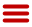# Find How to Assign Weights to Interfaces

If you use Fireware with a Pro upgrade, you can assign a weight to each interface used in your round-robin multi-WAN configuration. By default, each interface has a weight of 1. The weight refers to the proportion of load that the Firebox sends through an interface.

You can use only whole numbers for the interface weights; no fractions or decimals are allowed. For optimal load balancing, you might have to do a calculation to know the whole-number weight to assign for each interface. Use a common multiplier so that the relative proportion of the bandwidth given by each external connection is resolved to whole numbers.

For example, suppose you have three Internet connections. One ISP gives you 6 Mbps, another ISP gives you 1.5 Mbps, and a third gives you 768 Kbps. Convert the proportion to whole numbers:

• First convert the 768 Kbps to approximately .75 Mbps so that you use the same unit of measurement for all three lines. Your three lines are rated at 6, 1.5, and .75 Mbps.
• Multiply each value by 100 to remove the decimals. Proportionally, these are equivalent: [6 : 1.5 : .75] is the same ratio as [600 : 150 : 75]
• Find the greatest common divisor of the three numbers. In this case, 75 is the largest number that evenly divides all three numbers 600, 150, and 75.
• Divide each of the numbers by the greatest common divisor.

The results are 8, 2, and 1. You could use these numbers as weights in a round-robin multi-WAN configuration.